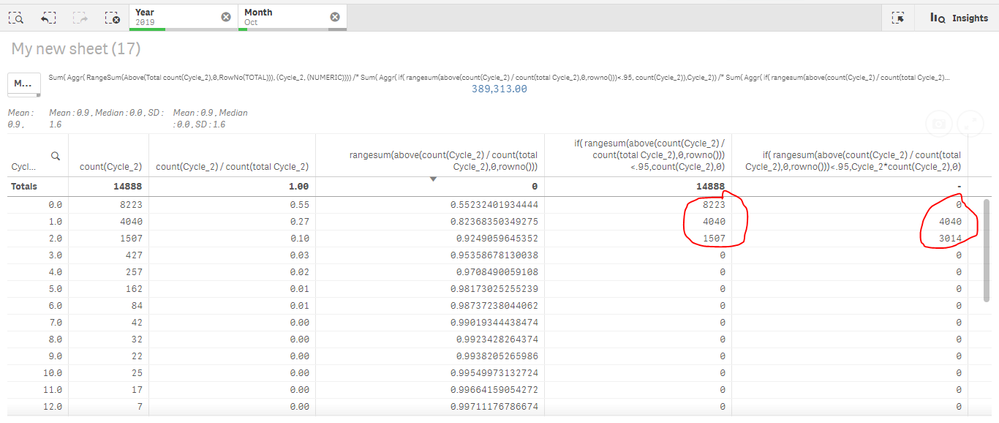# Qlik Sense App Development

Announcements
Coming your way, the Qlik Data Revolution Virtual Summit. October 27-29. REGISTER
cancel
Showing results for
Did you mean:
HighlightedSpecialist

## How to write the expression in KPI

Hello All ,

From the below image , i need to show  the last two columns  cumulative sum in two different KPI ,

KPI1:  sum(8223+4040+1507)

KPI2: sum(0+4040+3014)

Selection Year,Month

Dimension:Cycle_2

expression (Last but one cloumn):

if(
rangesum(above(count(Cycle_2)
/
count(total Cycle_2),0,rowno()))<.95,count(Cycle_2),0)

,

Expression(Last Column) :

if(
rangesum(above(count(Cycle_2)
/
count(total Cycle_2),0,rowno()))<.95,Cycle_2*count(Cycle_2),0)Thanks

1 Solution

Accepted Solutions
HighlightedMVP

What is the dimension of your chart below? Is it called Cycle_2? If it is, then try something like this

``````Sum(Aggr(

If(RangeSum(Above(Count(Cycle_2)/Count(TOTAL Cycle_2), 0, RowNo())) < 0.95, Count(Cycle_2), 0)

, (Cycle_2, (NUMERIC))))``````
2 Replies
HighlightedMVP

What is the dimension of your chart below? Is it called Cycle_2? If it is, then try something like this

``````Sum(Aggr(

If(RangeSum(Above(Count(Cycle_2)/Count(TOTAL Cycle_2), 0, RowNo())) < 0.95, Count(Cycle_2), 0)

, (Cycle_2, (NUMERIC))))``````
HighlightedSpecialist

Thanks Sunny,It is working